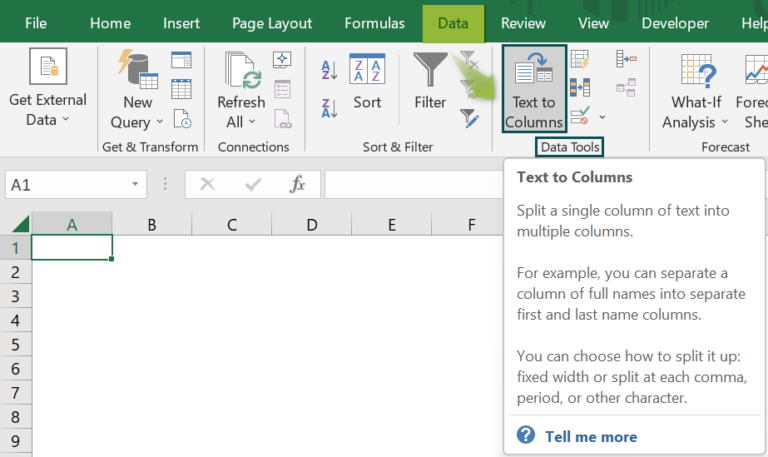# Separate Text in ExcelArticle byExcelMojo Team## What Is Separate Text In Excel?

The Separate Text in Excel is a feature that distributes the texts in different cells using spaces, commas, words, etc., using Text to Columns in excel option available under Data Tools in the Data tab.

For example, the succeeding image shows the text in cell A2. Now, we will Separate the Text between cells B2 and C2 using the Text to Columns in Excel.

The result is shown in cells B2 as ‘Jini’ and C2 as ‘Jones.’

Likewise, we can use separate text in excel feature.

###### Key Takeaways
• Splitting of text without any special characters can be done manually.
• The Separation of Text requires specific characters, certain methods, and techniques.
• The TEXTBEFORE function and text on the right side with the TEXTAFTER function are also used.
• If the data in the column doesn’t follow a standard format, for instance, if some names include extra data, then, excel splits that extra data into extra columns.
• We can import any spreadsheet into Google Sheets or make a new spreadsheet and paste in the data we want to split.

### Separate Text In Excel Shortcut

The shortcut keys to Separate Text in Excel are;

To select the cells which we want to split, press,

ALT key + A for Data tab + E for Splitting Text

### How To Use Separate Text In Excel?

For example, the succeeding image shows an idiom as a string in column A. Now, we will Separate Text between multiple columns using the Text to Columns in Excel with a space delimiter.

The steps to Splitting the Single data between multiple columns are as follows:

1. To begin with, select the cells which we want to split. In this case, the selected cell is cell A2.

2. Next, go to the Data tab.

3. Then, select the Text to Columns option from the Data Tools group.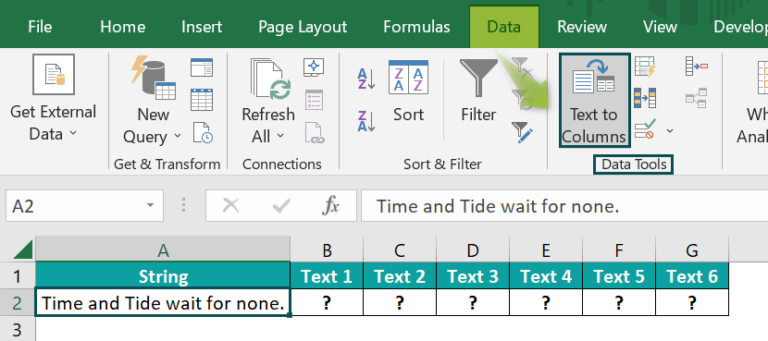4. Next, the window Convert Text to Columns Wizard – Step 1 of 3 opens.

5. Now, select the Delimited radio button from the Original data type list.

6. Click Next.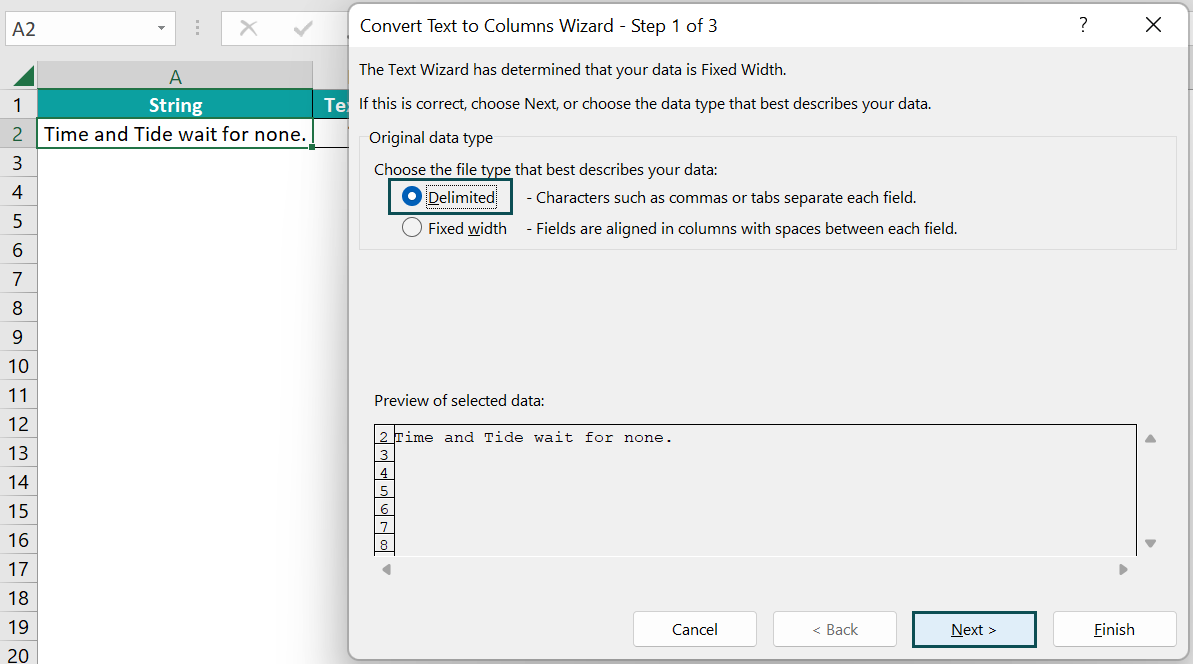7. Next, the window Convert Text to Columns Wizard – Step 2 of 3 opens.

8. Now, select the Space checkbox from the Delimiters list.

9. Click Next.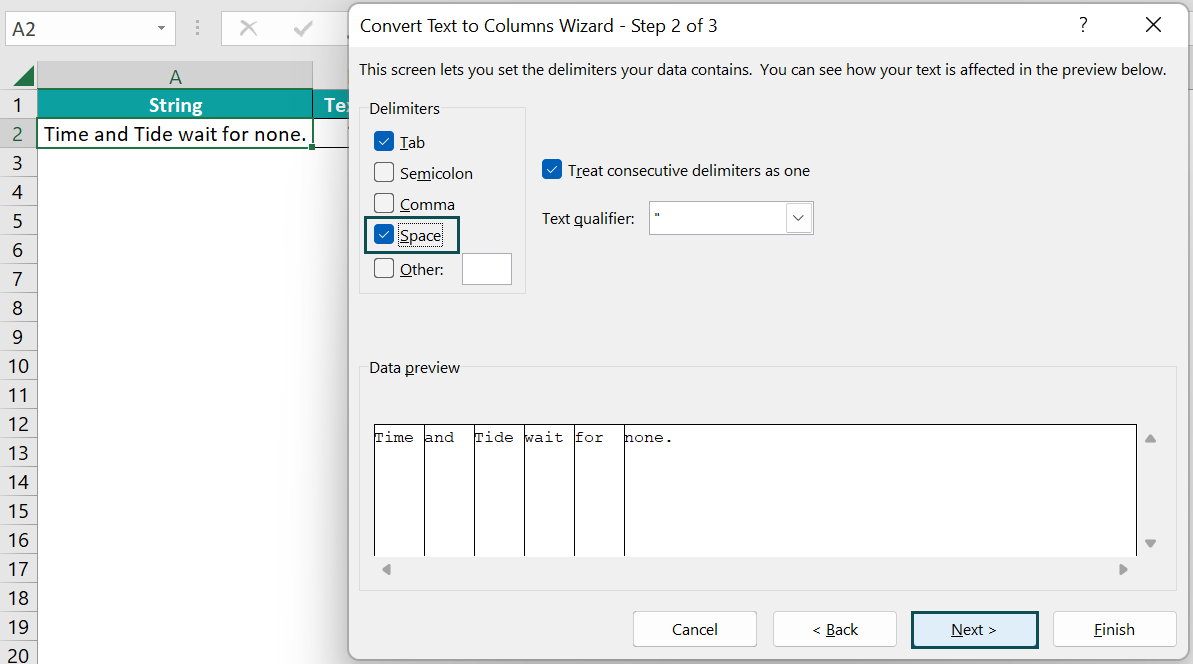10. Next, the window Convert Text to Columns Wizard – Step 3 of 3 opens.

11. Now, select the General radio button from the Column data format list.

12. Then, set the cell reference where we want to print the result in the Destination box. In this case, the cell reference will be \$B\$2.

13. Finally, click Finish.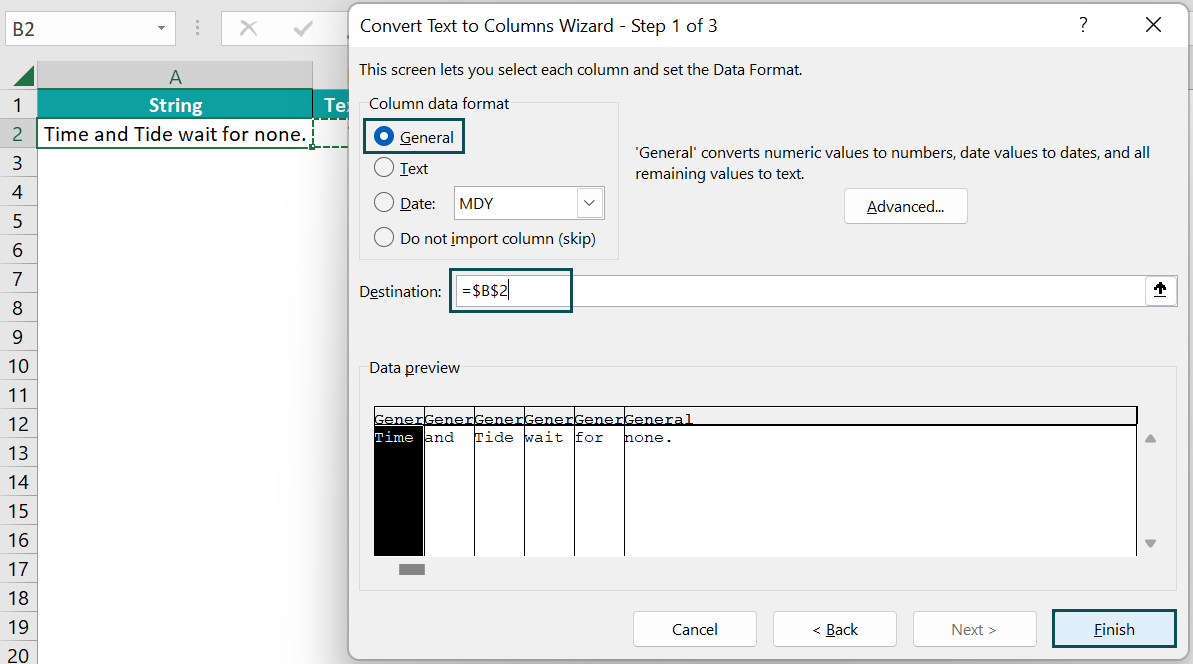14. The result is displayed in the cells B2, C2, D2, E2, F2, and G2, as the string Time and Tide wait for none. is split as Time, and, Tide, wait, for, and none.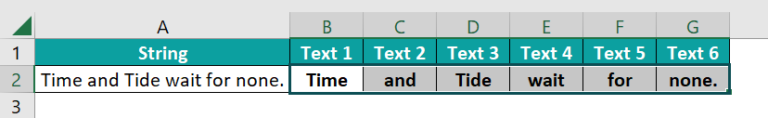Likewise, we can use separate text in excel feature.

### Examples

#### Example #1

For example, the succeeding image shows two fruits’ names separated with a comma and space in column A. Now, we will Separate Text between two columns using the Text to Columns in Excel with a comma and space delimiter.

The steps to Splitting the Single data between multiple columns are as follows:

• Step 1: To begin with, select the cells which you want to split. In this case, the selected cell is cell A2.
• Step 2: Then, go to the Data tab.
• Step 3: Now, select the Text to Columns option from the Data Tools group.
• Step 4: Next, the window Convert Text to Columns Wizard – Step 1 of 3 opens.
• Step 5: Now, select the Delimited radio button from the Original data type list.
• Step 6: Click Next.
• Step 7: Next, the window Convert Text to Columns Wizard – Step 2 of 3 opens.
• Step 8: Then, select the Comma and Space checkboxes from the Delimiters list.
• Step 9: Click Next.
• Step 10: The window Convert Text to Columns Wizard – Step 3 of 3 opens.
• Step 11: Next, select the General radio button from the Column data format list.
• Step 12: Then, set the excel cell reference where we want to print the result in the Destination box. In this case, the cell reference will be \$B\$2.
• Step 13: Click Finish.
• The result is displayed in cells B2 and C2.

Similarly, we can use separate text in excel option to separate data.

#### Example #2

For example, the succeeding image shows the date and time in column A. Next, we will Separate Text between two columns using Text to Columns in Excel with a fixed width method.

The steps used to split the single data between multiple columns are as follows:

• Step 1: First, select the cells which we want to split. In this case, the selected cell is cell A2.
• Step 2: Then, go to the Data tab.
• Step 3: Now, select the Text to Columns option from the Data Tools group.
• Step 4: Next, the window Convert Text to Columns Wizard – Step 1 of 3 opens.
• Step 5: Then, select the Fixed width radio button from the Original data type list.
• Step 6: Click Next.
• Step 7: Next, the window Convert Text to Columns Wizard – Step 2 of 3 opens.
• Step 8: Now, this page shows that if we want to adjust the break line, we can do so.
• Step 9: Then, click Next.
• Step 10: Next, the window Convert Text to Columns Wizard – Step 3 of 3 opens.
• Step 11: Then, select the General radio button from the Column data format list.
• Step 12: Now, set the cell reference where we want to print the result in the Destination box. In this case, the cell reference will be \$B\$2.
• Step 13: Finally, click Finish.
• Step 14: The result is displayed in cells B2 and C2 as shown in the below image.

Likewise, we can make use of separate text in excel option.

#### Example #3

The TRANSPOSE function can move the Text from Columns to rows in Excel. It transposes the column’s data into a row and vice versa.

For example, the following image shows the array of two numbers, “10 and 20,” as input in Row 2. Next, we must transpose the data table into the column with the TRANSPOSE formula.

The steps to calculate the value by the TRANSPOSE Excel Function are as follows:

• Step 1: First, select the cell where we will enter the formula and calculate the result. The selected cell, in this case, are cells D2 and D3.
• Step 2:  Next, we will enter the Formula of the TRANSPOSE Excel Function in range D2:D3.
• Step 3: Now, enter the value of ‘array’ as A2:B2, i.e., move the Text from Columns to rows in Excel
• Step 4: The entered complete formula is {=TRANSPOSE(A2:B2)} in cell D2:D3.
• Step 5: Next, press the Enter key. The results in cells D2 and D3 are 10 and 20, respectively. Now, we can see the results in the column as a vertical representation, as shown in the image below.

Similarly, we can use separate text in function to separate data in excel.

### Important Things To Note

• The Fixed-Width method is used in splitting data based on the length of the character.
• The Delimited method is used in splitting the data based on a set of delimiters such as commas, semicolons, tabs, etc.
• For Separate Text in Excel, we use the Text to Column feature of Excel.
• Similarly, the CONCATENATE function is used to concatenate the texts.
• The Separate Text Keyboard shortcuts in excel are ALT + A + E.
• The Separate Text can convert the date formats.
• It can convert numbers with trailing minus signs to negative numbers.

1. What does Separate Text do in Excel?

The Separate Text in Excel separates a text into different columns based on delimited or fixed width. There are two ways to split Text into Columns in Excel. The first uses a delimiter in which we provide input as a comma, space, or hyphen; the second is a fixed defined width to separate text in the adjacent columns.

2. How to remove Separate Text in Excel?

We can remove Separate Text in Excel using CONCATENATE function under More Functions > Compatibility option > Function Library > Formula tab. It merges the selected cells into one cell.

For example, the succeeding image shows the first name in column A and the last word in column B. Now, we will remove the Separate Text in Excel using CONCATENATE function.

The steps to calculate the value by the CONCATENATE Excel Function are as follows: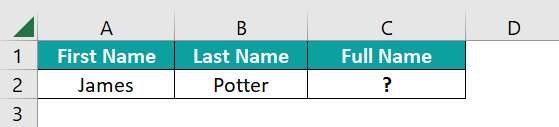• Step 1: First, select the cell where we will enter the formula and then, calculate the result. The selected cell, in this case, is cell C2.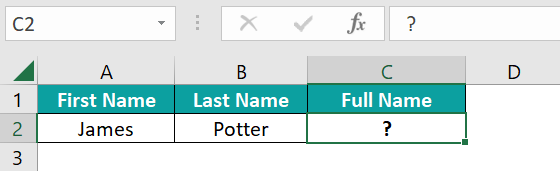• Step 2:  Next, we will enter the Formula of the CONCATENATE Excel Function in cell C2.

• Step 3: Then, enter the value of text1 as A2, i.e., the first text

• Step 4: Now, enter the value of text2 as “ ”, i.e., the second text is space

• Step 5: Simialrly, enter the value of text3 as B2, i.e., the third text

• Step 6: So, the entered complete formula is =CONCATENATE(A2,“ ”,B2) in cell C2.

• Step 7: Finally, press the Enter key. Now, we will obtain the results as shown in the image below.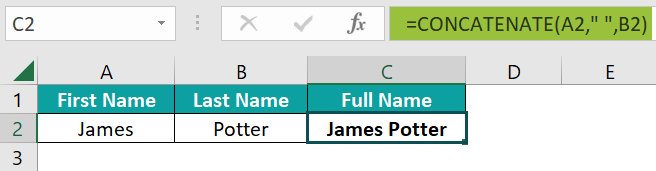3. Where to find Text to Columns option in Excel?

One can activate the Text to Column option in Excel using the following steps:

1) First, select the cell which will contain the result.
2) Next, go to the Data tab.
3) Then, click on the Text to Columns option from the Data Tools group.